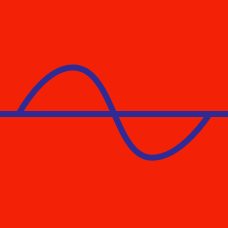Geometry

Trigonometric Graphs - Amplitude and Periodicity

Consider two functions $f(x)=25-\sin 6x, g(x)=\left\lfloor \frac{x}{2} \right\rfloor.$ Find the sum of all the elements in the range of $g(f(x))$.

Details and assumptions

The function $\lfloor x \rfloor: \mathbb{R} \rightarrow \mathbb{Z}$ refers to the greatest integer smaller than or equal to $x$. For example, $\lfloor 2.3 \rfloor = 2$ and $\lfloor -5 \rfloor = -5$.

If the period of the function $f(x)=\sqrt{224+\cos x}+\sqrt{226-\cos x}$ is $a$, what is the value of $f(a)$?

Consider two functions $f(x)=7\sin x+12, g(x)=\pi\cos \pi x.$ If $a$ and $b$ are the maximum and minimum values, respectively, of $f(g(x))$, what is $a+b$?

The graph of $y=7 \tan (2\pi x-\pi)+18$ has period $\frac{1}{a}$. What is the value of $a$?

What is the sum of the maximum and minimum values of the function $y=|\sin x-7|+20?$

×